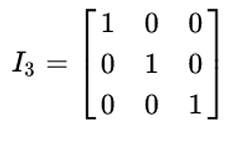# Print the identity matrix using numpy.eye() function | Linear Algebra using Python

Linear Algebra using Python | numpy.eye() function: Here, we are going to learn how to print the identity matrix using numpy.eye() function in Python?
Submitted by Anuj Singh, on May 25, 2020

Prerequisite:

In linear algebra, the identity matrix, of size n is the n × n square matrix with ones on the main diagonal and zeros elsewhere. It is denoted by I. Also known as the unit matrix because its determinant value is 1 irrespective of size. This is the key feature of an identity matrix and it plays an important role in Linear Algebra.Syntax:

```    identity_matrix = eye(n)
```

Input parameter(s):

• n – size of the identity matrix.

Return value:

A square matrix with 1 at its diagonal and rest is zero.

Applications:

1. Machine Learning
2. Neural Network
3. Linear Algebra - Gauss Jordan Elimination

## Python code to print the identity matrix using numpy.eye() function

```# Linear Algebra Learning Sequence
# Identity matrix using numpy.eye() function

import numpy as np

# Using power function
print("\n---I(4x4)---\n", np.eye(4))
print("\n---I(6x6)---\n", np.eye(6))
```

Output:

```---I(4x4)---
[[1. 0. 0. 0.]
[0. 1. 0. 0.]
[0. 0. 1. 0.]
[0. 0. 0. 1.]]

---I(6x6)---
[[1. 0. 0. 0. 0. 0.]
[0. 1. 0. 0. 0. 0.]
[0. 0. 1. 0. 0. 0.]
[0. 0. 0. 1. 0. 0.]
[0. 0. 0. 0. 1. 0.]
[0. 0. 0. 0. 0. 1.]]
```

Preparation

What's New

Top Interview Coding Problems/Challenges!